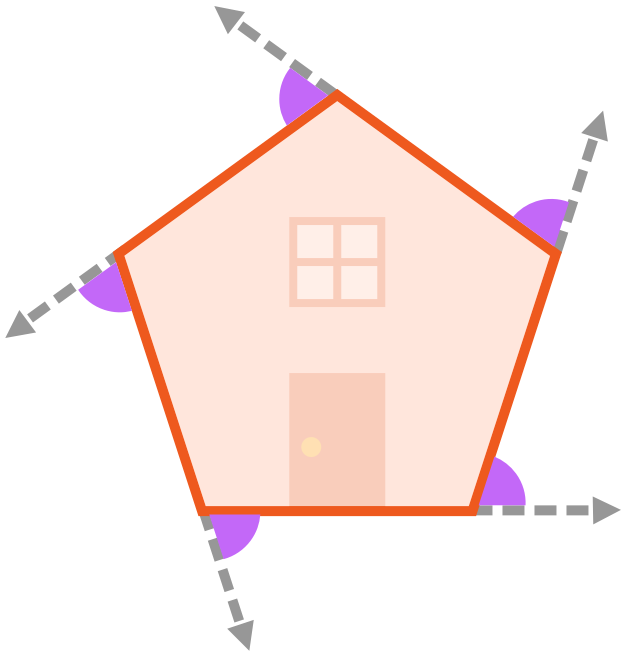•• Merch
• Donate
• SAT Prep

IntroductionIntroduction# Interior and Exterior Angles of Polygons

INTRO
The interior angles of a polygon are angles inside the shape. The exterior angles of a polygon are angles outside of the shape formed between any side of the polygon and a line extended from the side next to it.
If we imagine the polygon as a house, the interior angles live inside of the house, while the exterior angles live in exile outside of the house.CALCULATOR

## Interior & Exterior Angles Calculator

### Step 1. Determine whether you’re looking for interior or exterior angles.

If we think of the shape as a house, the interior angles live inside of the house, while the exterior angles live in exile outside of the house.Pick which type of angles you’re looking for.
KEY STEPS

## How to Find the Interior & Exterior Angles of Polygons

### Step 1. Determine whether you’re looking for interior or exterior angles.

If we imagine the polygon as a house, the interior angles live inside of the house, while the exterior angles live in exile outside of the house.Type of Angles:

### Step 4. Find the value of an individual angle.

If your shape is regular, just divide the sum of the interior angles by the number of sides/angles:
If your shape is irregular and you have the values of all the other interior angles, you can find the missing angle by subtracting your given angles from the sum:
LESSON
Interior Angles

## Interior Angles

The interior angles of a polygon are angles inside the shape formed at the points where the sides connect. If we imagine the polygon as a house, the interior angles live inside the house.We can find the sum of a polygon’s interior angles by using the number of sides the shape has:
PRACTICE
Interior Angles

## Practice: Interior Angles

Question 1 of 6:Find the value of an interior angle in a regular 6-sided shape.

### Step 1. Determine whether you’re looking for an interior or exterior angle.

Are we looking for an interior or exterior angle?

LESSON
Exterior Angles

## Exterior Angles

The exterior angles of a polygon are angles outside of the shape formed between any side of the polygon and a line extended from the next side.
If we imagine the polygon as a house, the exterior angles live in exile outside of the house.As we decrease the size of any polygon, we can easily see that the exterior angles add up to one full rotation of .
Number of sides:This is true for all polygons, which means the sum of exterior angles for all polygons is always .
PRACTICE
Exterior Angles

## Practice: Exterior Angles

Question 1 of 6:Find the value of an exterior angle in a regular 6-sided shape.

### Step 1. Determine whether you’re looking for an interior or exterior angle.

Are we looking for an interior or exterior angle?

CONCLUSION
Dang, look at you go! Thanks for checking out this lesson ☺️🙏. Where to next?Leave Feedback